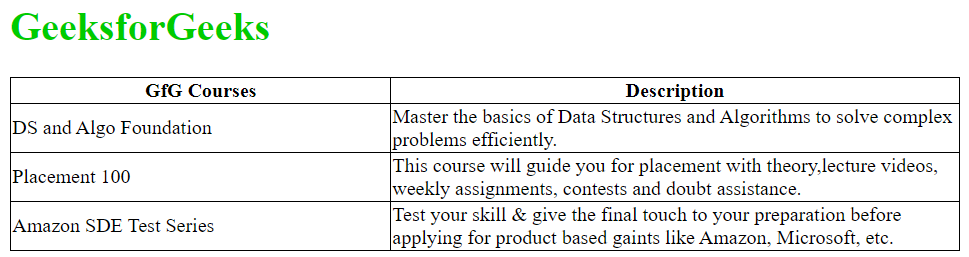# How to set fixed width for <td> in a table ?

• Difficulty Level : Easy
• Last Updated : 19 May, 2022

The requirement of a table is very normal in the process of designing a web page. HTML provides the <table> tag to construct the table and to define rows and columns <tr> and <td> tags respectively are used.

By default, the dimensions of the rows and columns in a table are adjusted by the browser automatically in a way that fits the contents. However, there can be situations where the width of the columns are needed to be fixed. There are multiple ways to fix the width for <td> tag. Some of them are mentioned below:

• Using width attribute: The <td> tag has width attribute to control the width of a particular column. By assigning a numeric value to this attribute between 0 to 100 in terms of percentage(or you can use pixel format). We can restrict the column width up to that much percentage of the table’s total width.  The width attribute is invalid and has been disapproved, thus it is no longer supported by HTML5.

## HTML

 ```<``html``>``    ``<``head``>``        ``<``title``>``          ``Set up fixed width for``        ````        ``<``meta` `charset``=``"UTF-8"` `/>``        ``<``meta` `name``=``"viewport"``              ``content="``width``=``device``-width,``                       ``initial-scale``=``1``.0" />` `        ``<``style``>``            ``table,``            ``th,``            ``td {``                ``border: 1px solid black;``                ``border-collapse: collapse;``            ``}``        ````    ````    ``<``body``>``        ``<``h1` `style``=``"color: #00cc00;"``>``         ``GeeksforGeeks``        ``` `        ````        ``<``div` `style``=``"overflow-x: auto;"``>``          ` `            ````            ``<``table` `width``=``"50%"``>``                ``<``tr``>``                    ``<``th``>GfG Courses``                    ``<``th``>Description``                ````                ``<``tr``>``                    ``<``td` `width``=``"40%"``>``                      ``DS and Algo Foundation``                    ````                    ``<``td` `width``=``"60%"``>``                      ``Master the basics of Data Structures``                      ``and Algorithms to solve complex``                      ``problems efficiently.``                    ````                ````                ``<``tr``>``                    ``<``td` `width``=``"40%"``>Placement 100``                    ``<``td` `width``=``"60%"``>``                      ``This course will guide you for``                      ``placement with theory,lecture videos,``                      ``weekly assignments, contests and doubt``                      ``assistance.``                    ````                ````                ``<``tr``>``                    ``<``td` `width``=``"40%"``>``                      ``Amazon SDE Test Series``                    ````                    ``<``td` `width``=``"60%"``>``                      ``Test your skill & give the final``                      ``touch to your preparation before``                      ``applying for product based against``                      ``like Amazon, Microsoft, etc.``                    ````                ````            ````        ````    `````

• Using CSS: The Cascading Style Sheet(CSS) is widely used to decorate the web pages which are of vast sizes. By using CSS, the styling of HTML elements is easy to modify. To fix the width of td tag the nth-child CSS is used to set the property of specific columns(determined by the value of n) in each row of the table.

## HTML

 ```<``html``>``    ``<``head``>``        ``<``title``>Set up fixed width for <``td``>``        ``<``meta` `charset``=``"UTF-8"` `/>``        ``<``meta` `name``=``"viewport"``              ``content="``width``=``device``-width,``                       ``initial-scale``=``1``.0" />` `        ``<``style``>``            ``table,``            ``th,``            ``td {``                ``border: 1px solid black;``                ``border-collapse: collapse;``            ``}` `            ``table {``                ``width: 50%;``            ``}` `            ``// Fixing width of first``            ``// column of each row``            ``td:nth-child(1) {``                ``width: 40%;``            ``}` `            ``// Fixing width of second``            ``// column of each row``            ``td:nth-child(2) {``                ``width: 60%;``            ``}``        ````    ````    ``<``body``>``        ``<``h1` `style``=``"color: #00cc00;"``>``         ``GeeksforGeeks``        ``` `        ````        ``<``div` `style``=``"overflow-x: auto;"``>``          ` `            ````            ``<``table``>``                ``<``tr``>``                    ``<``th``>GfG Courses``                    ``<``th``>Description``                ``` `                ``<``tr``>``                    ``<``td``>DS and Algo Foundation``                    ``<``td``>``                       ``Master the basics of Data Structures``                       ``and Algorithms to solve complex``                       ``problems efficiently.``                    ````                ``` `                ``<``tr``>``                    ``<``td``>Placement 100``                    ``<``td``>``                     ``Test your skill & give the final touch``                     ``to your preparation before applying for``                     ``product based against like Amazon,``                     ``Microsoft, etc.``                    ````                ````                ``<``tr``>``                    ``<``td``>Amazon SDE Test Series``                    ``<``td``>``                     ``Test your skill & give the final touch``                     ``to your preparation before applying for``                     ``product based against like Amazon,``                     ``Microsoft, etc.``                    ````                ````            ````        ````    `````

• Using col tag and fixing the table layout property: If the same kind of column property is to be followed in every row of a table then using col tag to define the properties of the column is a great idea. If the col tag is written in the HTML document for the first time and its attributes are set, those all property refers to the first column of each row of the table inside which it is mentioned. Similarly using col tag for the second time and defining its attributes make its impact on the second column of each row of the table. Moreover, to adjust the long texts inside the columns CSS property table-layout:fixed; is used. Below is the implementation.

## HTML

 ```<``html``>``    ``<``head``>``        ``<``title``>Set up fixed width for``        ``<``meta` `charset``=``"UTF-8"` `/>``        ``<``meta` `name``=``"viewport"``              ``content="``width``=``device``-width,``                       ``initial-scale``=``1``.0" />` `        ``<``style``>``            ``table,``            ``th,``            ``td {``                ``border: 1px solid black;``                ``border-collapse: collapse;``            ``}` `            ``table {``                ``width: 50%;``            ``}` `            ``table.fixed {``                ``table-layout: fixed;``            ``}``            ``table.fixed td {``                ``overflow: hidden;``            ``}``        ````    ````    ``<``body``>``        ``<``h1` `style``=``"color: #00cc00;"``>``         ``GeeksforGeeks``        ``` `        ````        ``<``div` `style``=``"overflow-x: auto;"``>``            ````            ``<``table``>``                ````                ``<``col` `style``=``"width: 40%;"` `/>` `                ````                ``<``col` `style``=``"width: 60%;"` `/>` `                ``<``tr``>``                    ``<``th``>GfG Courses``                    ``<``th``>Description``                ``` `                ``<``tr``>``                    ``<``td``>DS and Algo Foundation``                    ``<``td``>``                      ``Master the basics of Data Structures``                      ``and Algorithms to solve complex``                      ``problems efficiently.``                    ````                ``` `                ``<``tr``>``                    ``<``td``>Placement 100``                    ``<``td``>``                      ``This course will guide you for``                      ``placement with theory,lecture``                      ``videos, weekly assignments,``                      ``contests and doubt assistance.``                    ````                ``` `                ``<``tr``>``                    ``<``td``>Amazon SDE Test Series``                    ``<``td``>``                     ``Test your skill & give the final touch``                     ``to your preparation before applying for``                     ``product based against like Amazon,``                     ``Microsoft, etc.``                    ````                ````            ````        ````    `````

• Output: The output will be same for every method.HTML is the foundation of webpages, is used for webpage development by structuring websites and web apps.You can learn HTML from the ground up by following this HTML Tutorial and HTML Examples.

My Personal Notes arrow_drop_up